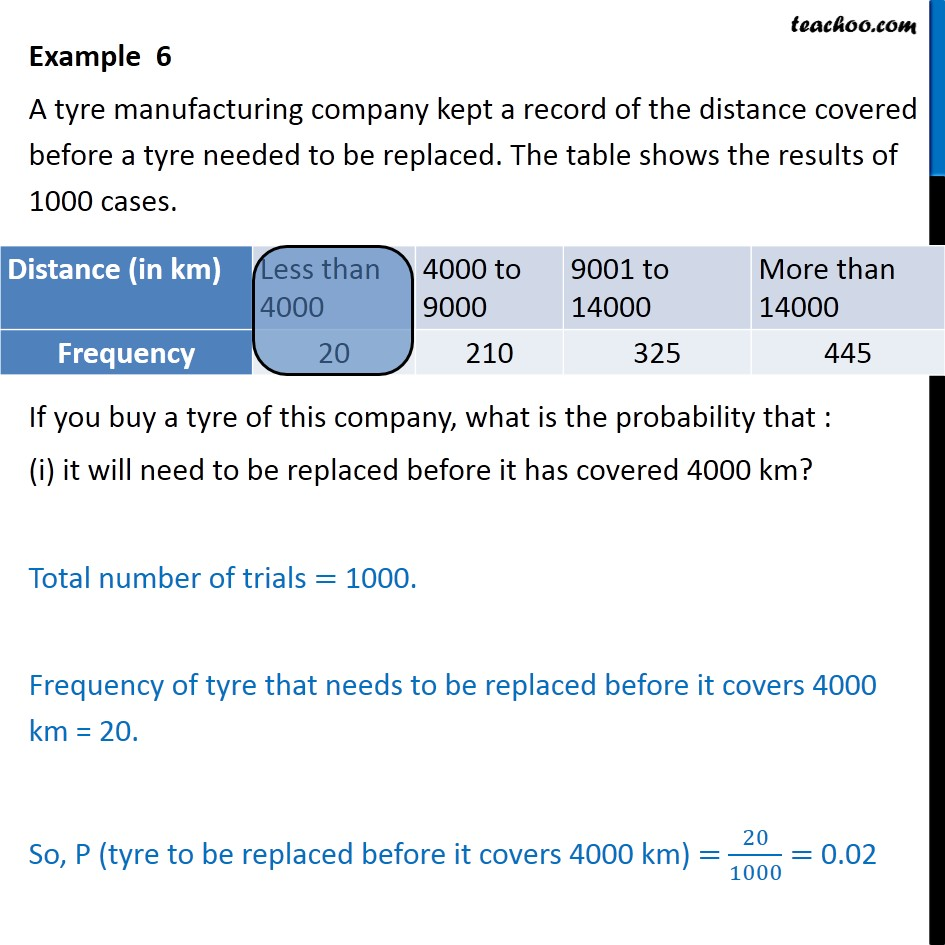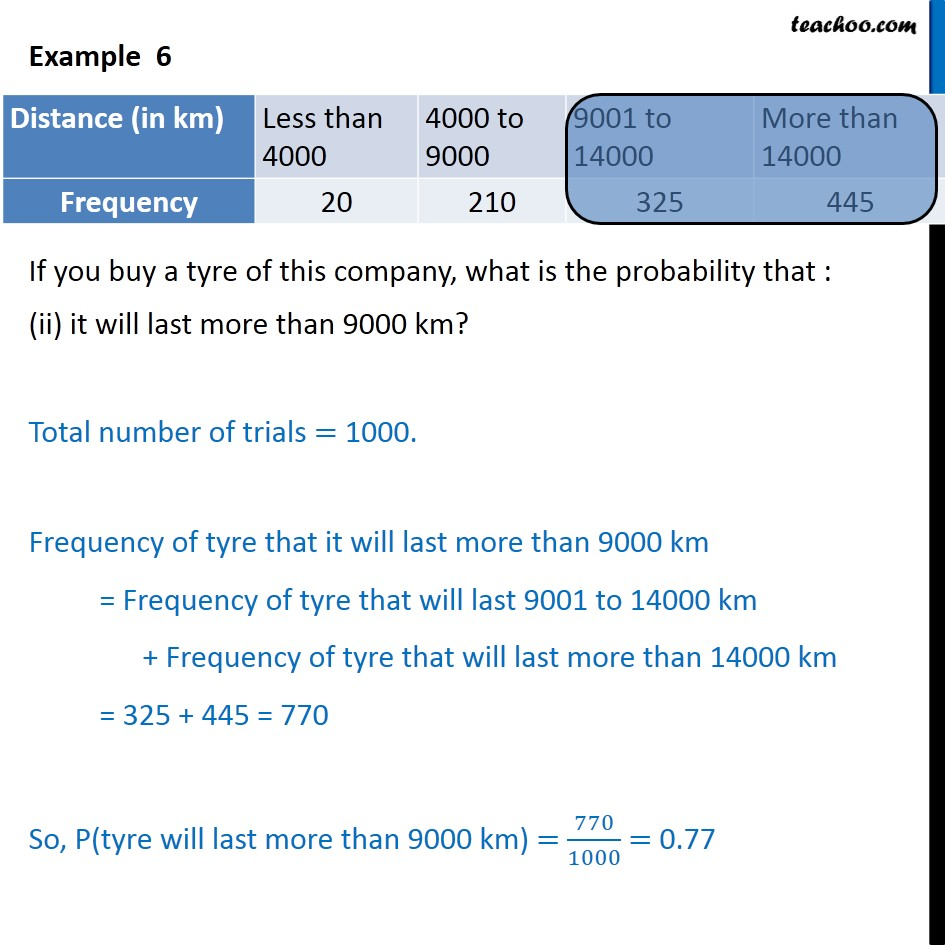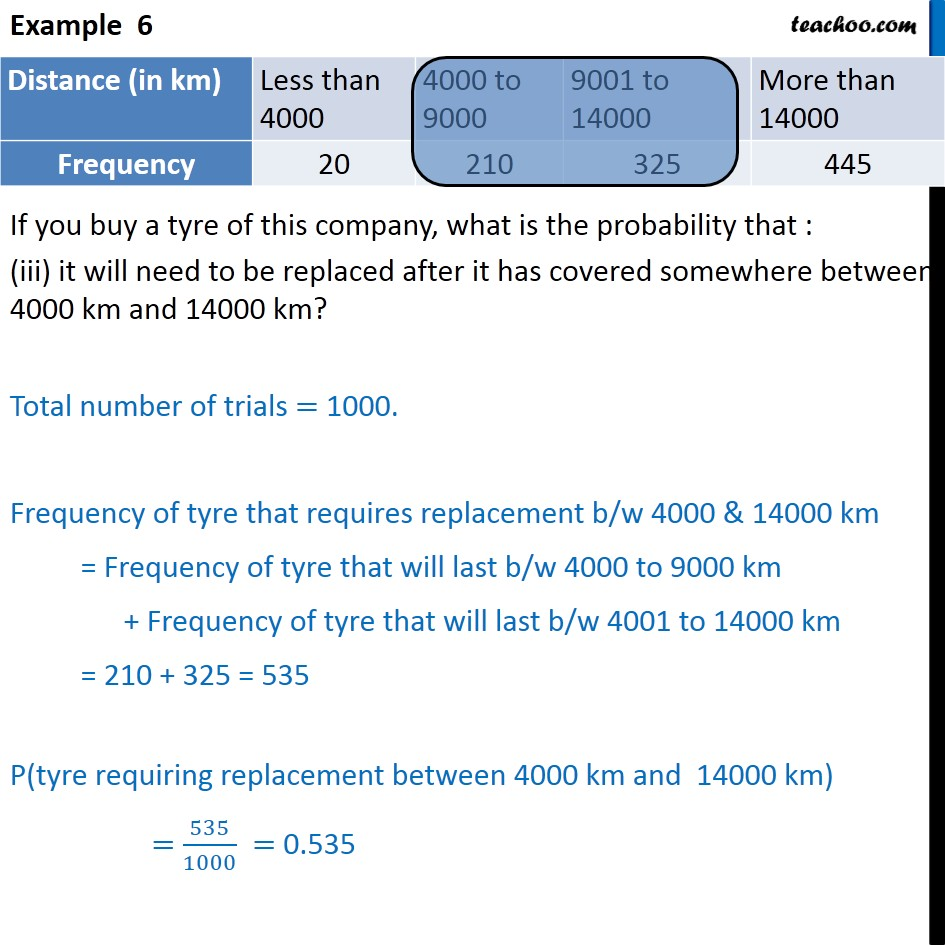Examples

Probability
Serial order wiseLearn in your speed, with individual attention - Teachoo Maths 1-on-1 Class

### Transcript

Example 6 A tyre manufacturing company kept a record of the distance covered before a tyre needed to be replaced. The table shows the results of 1000 cases. If you buy a tyre of this company, what is the probability that : (i) it will need to be replaced before it has covered 4000 km? Total number of trials = 1000. Frequency of tyre that needs to be replaced before it covers 4000 km = 20. So, P (tyre to be replaced before it covers 4000 km) = 20/1000 = 0.02 Example 6 If you buy a tyre of this company, what is the probability that : (ii) it will last more than 9000 km? Total number of trials = 1000. Frequency of tyre that it will last more than 9000 km = Frequency of tyre that will last 9001 to 14000 km + Frequency of tyre that will last more than 14000 km = 325 + 445 = 770 So, P(tyre will last more than 9000 km) = 770/1000 = 0.77 Example 6 If you buy a tyre of this company, what is the probability that : (iii) it will need to be replaced after it has covered somewhere between 4000 km and 14000 km? Total number of trials = 1000. Frequency of tyre that requires replacement b/w 4000 & 14000 km = Frequency of tyre that will last b/w 4000 to 9000 km + Frequency of tyre that will last b/w 4001 to 14000 km = 210 + 325 = 535 P(tyre requiring replacement between 4000 km and 14000 km) = 535/1000 = 0.535